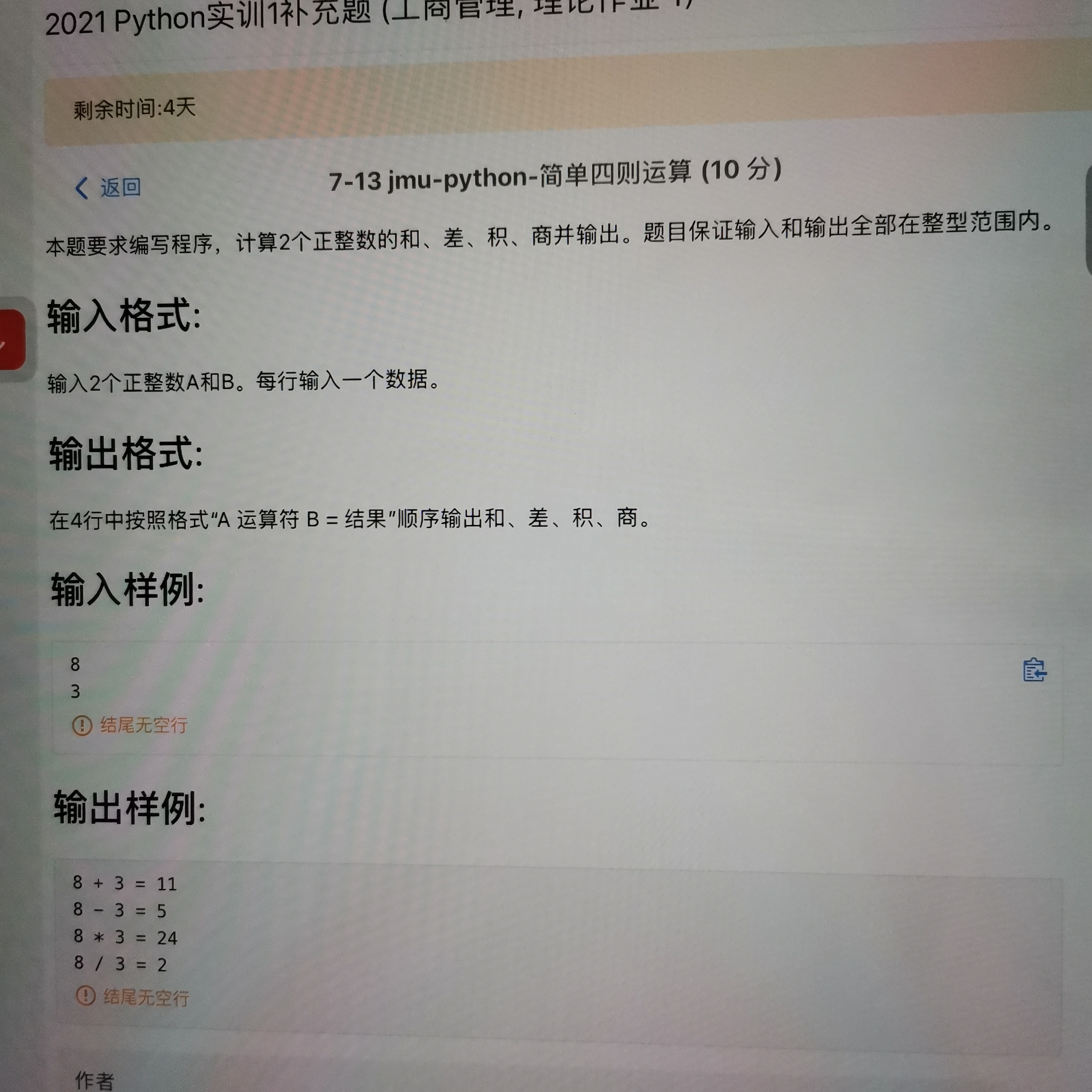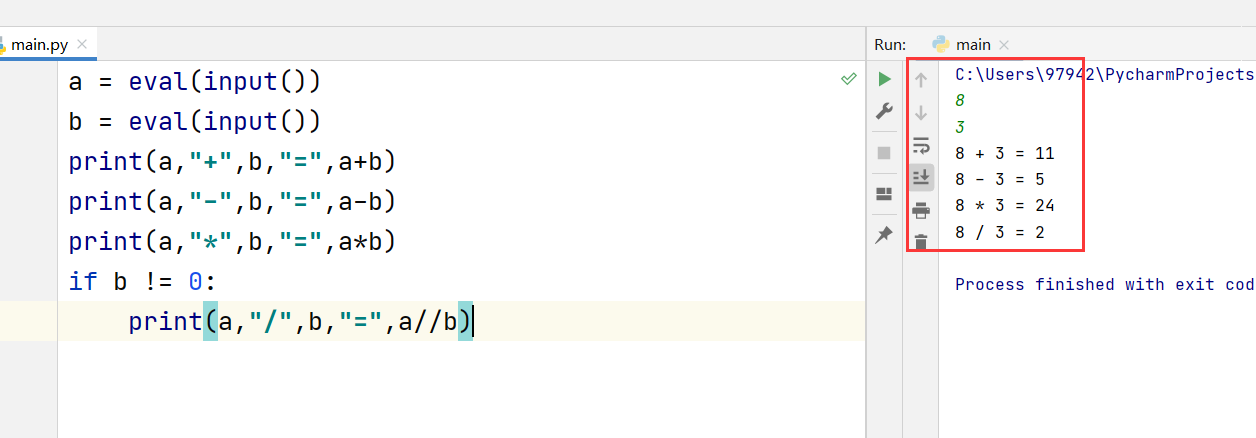2021-10-03 19:51

# 计算两个正整数的和差积商• 好问题 提建议
• 收藏

#### 1条回答默认 最新

•yangbocsu 2021-10-03 19:53
已采纳
``````
a = eval(input())
b = eval(input())
print(a,"+",b,"=",a+b)
print(a,"-",b,"=",a-b)
print(a,"*",b,"=",a*b)
if b != 0:
print(a,"/",b,"=",a//b)
``````已采纳该答案
评论
解决 无用
打赏 举报